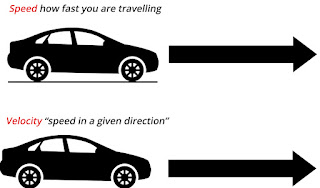## Social Items

### VELOCITY

The speed of a car (or any other body) gives us an idea of how fast the car is moving but it does not tell us the direction in which the car is moving. Thus, to know the exact position of a moving car we should also know the directioin in which the car is moving.
In other words, we should know the speed of the car as well as the direction of speed. This gives us another term known as velocity which can be defined as follows :  Velocity of a body is the distance travelled by it per unit time in a given direction. That is :

Velocity = Distance travelled in a given direction / Time taken

If  a body travels a distance 's' in time 't' in a given direction, then its velocity 'v' is given by :

v = s / t

where v = velocity of the body
s = distance travelled (in the given direction)
and  t = time taken (to travel that distance)

We know that the 'Distance Travelled in a given direction' is known as 'Displacement'. So, we can also write the difinition of velocity in terms of  'Displacement'. We can now say that : Velocity of a body is the displacement produced per unit time.  We can get the velocity of a body dividing the 'Diaplacement' by 'Time taken' for the displacement. Thus, we can write another formula for velocity. That is :
Velocity = Displacement / Time taken

v = s / t

where  v = velocity of the body
s = displacement of the body
and  t = time taken (for displacement)

### Read Also :

• The SI unit of velocity is the same as that of speed, namely, meters per second (or m/s).
• We can use the bigger unit of  kilometers per hour  to express the bigger values of velocities and centimetres per second  to express the small values of  velocities.
• It should be noted that both, speed as well as velocity, are represented by the same symbol v.
• The difference between speed and velocity is that speed has only magnitude (or size), it has no specific direction, but velocity has magnitude as well as direction.
• Speed is a scalar quantity (because it has magnitude only) but Velocity is a vector quantity (because it has magnitude as well as direction).
• The direction of velocity is the same as the direction od displacement of the body.
We have just seen that,  v = s / t

So, s = v × t

Thus, Distance travelled = Average velocity × Time

This formula should be memorized because it will be used in solving numerical problems.

If you like this article, do comment and share.## What is Velocity-Definition, Units, Formula, Examples and Equations

### VELOCITY

The speed of a car (or any other body) gives us an idea of how fast the car is moving but it does not tell us the direction in which the car is moving. Thus, to know the exact position of a moving car we should also know the directioin in which the car is moving.
In other words, we should know the speed of the car as well as the direction of speed. This gives us another term known as velocity which can be defined as follows :  Velocity of a body is the distance travelled by it per unit time in a given direction. That is :

Velocity = Distance travelled in a given direction / Time taken

If  a body travels a distance 's' in time 't' in a given direction, then its velocity 'v' is given by :

v = s / t

where v = velocity of the body
s = distance travelled (in the given direction)
and  t = time taken (to travel that distance)

We know that the 'Distance Travelled in a given direction' is known as 'Displacement'. So, we can also write the difinition of velocity in terms of  'Displacement'. We can now say that : Velocity of a body is the displacement produced per unit time.  We can get the velocity of a body dividing the 'Diaplacement' by 'Time taken' for the displacement. Thus, we can write another formula for velocity. That is :
Velocity = Displacement / Time taken

v = s / t

where  v = velocity of the body
s = displacement of the body
and  t = time taken (for displacement)

### Read Also :

• The SI unit of velocity is the same as that of speed, namely, meters per second (or m/s).
• We can use the bigger unit of  kilometers per hour  to express the bigger values of velocities and centimetres per second  to express the small values of  velocities.
• It should be noted that both, speed as well as velocity, are represented by the same symbol v.
• The difference between speed and velocity is that speed has only magnitude (or size), it has no specific direction, but velocity has magnitude as well as direction.
• Speed is a scalar quantity (because it has magnitude only) but Velocity is a vector quantity (because it has magnitude as well as direction).
• The direction of velocity is the same as the direction od displacement of the body.
We have just seen that,  v = s / t

So, s = v × t

Thus, Distance travelled = Average velocity × Time

This formula should be memorized because it will be used in solving numerical problems.

If you like this article, do comment and share.